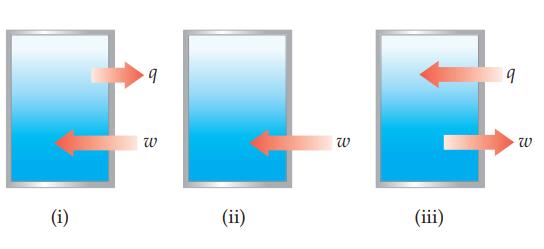×
Get Full Access to Chemistry: The Central Science - 14 Edition - Chapter 5 - Problem 5.4
Get Full Access to Chemistry: The Central Science - 14 Edition - Chapter 5 - Problem 5.4

×ISBN: 9780134414232 1274

## Solution for problem 5.4 Chapter 5

Chemistry: The Central Science | 14th Edition

• Textbook Solutions
• 2901 Step-by-step solutions solved by professors and subject experts
• Get 24/7 help from StudySoup virtual teaching assistantsChemistry: The Central Science | 14th Edition

4 5 1 288 Reviews
19
5
Problem 5.4

The contents of the closed box in each of the following illustrations represent a system, and the arrows show the changes to the system during some process. The lengths of the arrows represent the relative magnitudes of q and w.

(a) Which of these processes is endothermic?

(b) For which of these processes, if any, is $$\Delta E<0$$?

(c) For which process, if any, does the system experience a net gain in internal energy? [Section 5.2]Text Transcription:

\Delta E<0

Step-by-Step Solution:
Step 1 of 3

CHEM 103 Chapter 11 Notes Kinetic molecular theory of gases 1. Random, incessant motion (keeps on going around) 2. No attractive forces between gas molecules 3. Occupy a very small volume 4. Kinetic energy of gas molecules increases with increasing temperature ATM pressure ● Physics: Pressure = orce Area ● Pressure exerted by the column of air above us ● 14.7 psi (pounds per square inch) ○ Is equal to ■ 1 ATM (atmosphere) ■ 760 mm Hg ■ 760 torr ■ 101.325 Pascals Gases are described in terms of 4 properties ● Pressure (p) ● Volume (v) ● Temperature (t) ● Moles (n) ● Proportionality constant ( R ) ○ PV = nRT PV ○ n = ❑ R T❑ PV ❑ ○ R = at STP (Standard Temperature and Pressure) nT ❑ ■ STP Temperature is at 0 C or 273 K ■ STP Pressure is at 1 ATM ■ STP: 1 mole of any gas occupies 22.4 L 1mole 22.4L ● = 22.4L 1mole ● Example ○ Calculate gas constant R PV ❑ (1ATM)(22.4L) ❑ ATM∙L ■ R = R = = 0.0821 nT ❑ (1mole)(273K) mol∙K ● At STP, 1 mole occupies 22.4 L ○ How many grams of Helium are present in 8.00 L 8L

Step 2 of 3

Step 3 of 3

##### ISBN: 9780134414232

This full solution covers the following key subjects: . This expansive textbook survival guide covers 29 chapters, and 2820 solutions. The full step-by-step solution to problem: 5.4 from chapter: 5 was answered by , our top Chemistry solution expert on 10/03/18, 06:29PM. Chemistry: The Central Science was written by and is associated to the ISBN: 9780134414232. The answer to “?The contents of the closed box in each of the following illustrations represent a system, and the arrows show the changes to the system during some process. The lengths of the arrows represent the relative magnitudes of q and w. (a) Which of these processes is endothermic? (b) For which of these processes, if any, is $$\Delta E<0$$? (c) For which process, if any, does the system experience a net gain in internal energy? [Section 5.2] Text Transcription:\Delta E<0” is broken down into a number of easy to follow steps, and 79 words. This textbook survival guide was created for the textbook: Chemistry: The Central Science, edition: 14. Since the solution to 5.4 from 5 chapter was answered, more than 208 students have viewed the full step-by-step answer.

## Discover and learn what students are asking

Unlock Textbook Solution